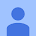Posts
Post has attachment
Finite Element Methods on Surfaces
In this post, we return to one of the roots of Nested Tori, namely, visualization of problems solved using finite element methods. There's quite a few projects that I worked on for that PhD, whose scattered results have not managed to make it on here today,...Post has attachment
The Best Cake
Optimization is one of the most useful tools in all of math. Many of the visualizations here based in finding the optimum solution to something (and indeed, it drives many of the solution methods of differential equations). Here's an optimization  puzzler b...Post has attachment
A Calculation in Statistical Mechanics
We've been harping about phase and state space for a long while, now, and have mentioned at more than one point that statistical mechanics is a subject dealing with, in some sense, the ultimate state space, of many gazillions of dimensions. It is amazing th...Post has attachment
The Volume of Intersecting Cylinders
Now for another classic, although not as "kewel" as the spiral example. This time, it was  I  who asked for the help: once upon a time in a calculus class, we were asked to compute the volume of the solid that is the intersection of two cylinders at right a...Post has attachment
Yitz..'s Kewel Spiral
Every once in a while, I get an "assignment" to calculate some interesting parametrization (because parametrizations are awesome like that). For example, the bowtie pasta was originally a request from a student. Today's goes way back, to someone asking a qu...Post has attachment
Marginalization of Asian roles in Hollywood continues ... Lay the smack down, George! #marginalizationjustsaynoPost has attachment
More Polar Classics: Spirals
Some more from the classic polar curves collection... today we specialize in spirals . The basic concept of what a spiral should be, in polar coordinates, is any plot $r$ varying with $\theta$, $r=f(\theta)$, where $f$ is always increasing, and $\theta$ is ...Variance Solids: Volumes and $\mathscr{L}^2$, Part 2
Last time , we talked about how the classical $\mathscr{L}^2$ norm of a (one-variable) function could be visualized as a solid consisting of squares. Of course, there's another formula that is defined by almost the same formula, with an extra factor of $\pi...Add a comment... Post has attachment Volumes of Solids and (squared)$\mathscr{L}^2$Norms The volume of this solid is the squared$\mathscr{L}^2$distance between$y=x^2$and$y=x^3$on the interval$[0,\frac{3}{2}]$. The good ol'$\mathscr{L}^2\$ norm has an outsized importance in many applications of math, principally, because Hilbert spaces (s...